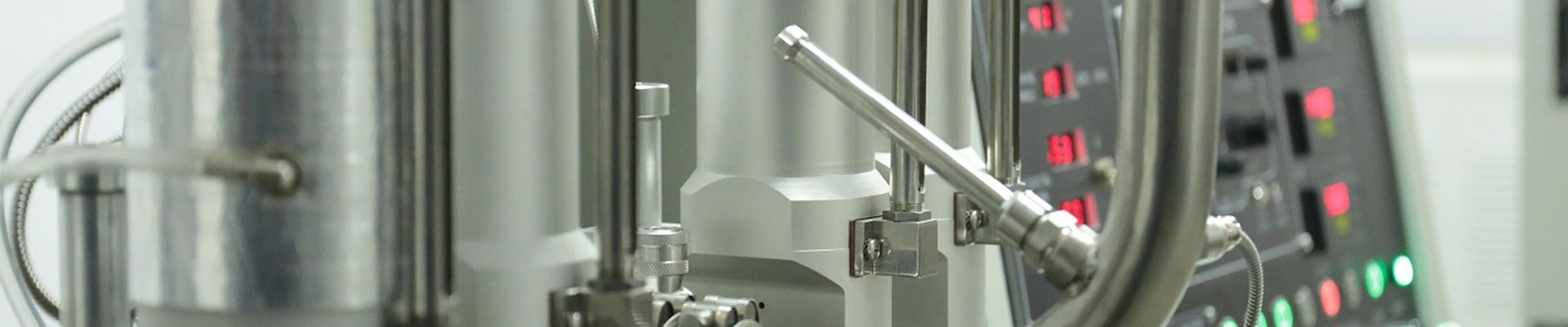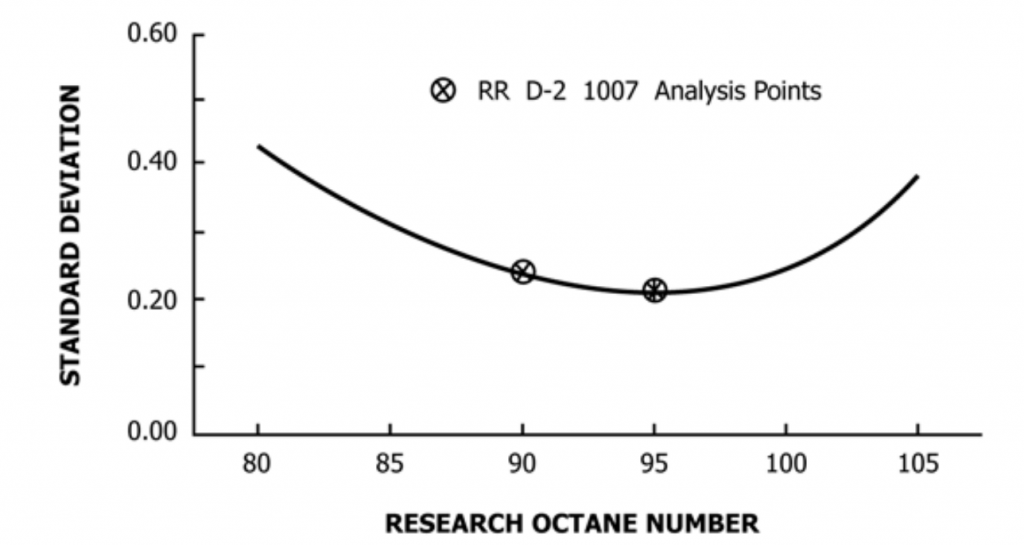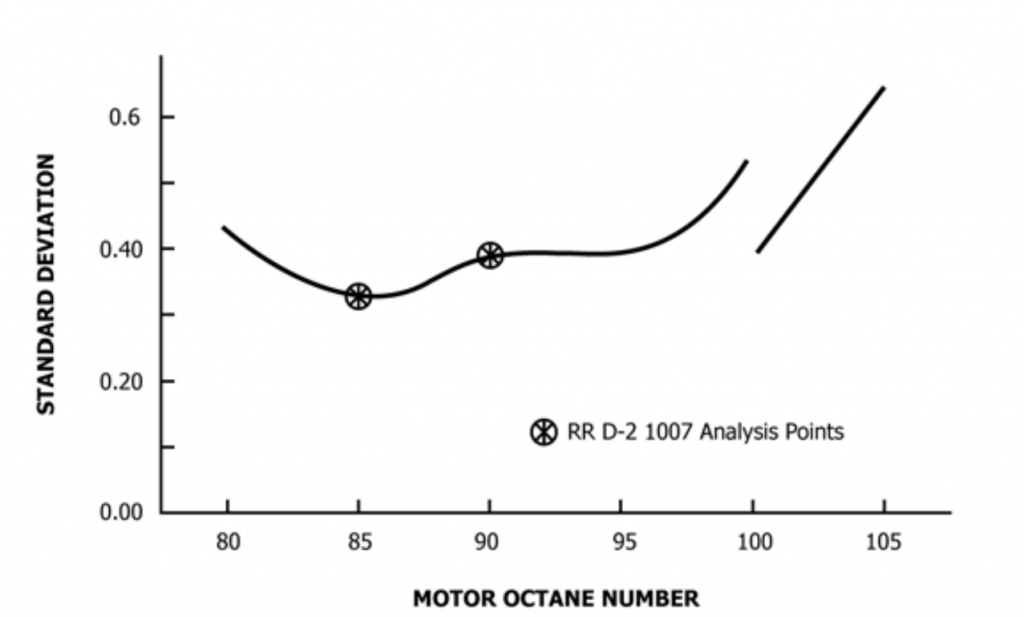En# Cfr Test Engine-RON/MON Octane Rating Precision and Bias

CFR test engine/SINPAR FTC RON&MON octane test engine is used as the most accurate way for testing the actual octane number of motor fuels, referred to as the “knock test engine“. It measures gasoline characteristics related to engine knocking, by Research Method (ASTM D2699 RON) and Motor Method (ASTM D2700 MON).

The RON/MON test method is to obtain the standard detonation strength, by changing the compression ratio and measuring the detonation strength with an electronic detonation meter.

The precision and bias of RON method (ASTM D2699) for the range of 90.0-100.0 octane number. That based on statistical examination of interlaboratory test results by the bracketing-equilibrium fuel level or C.R. procedures is as follows:

#### Repeatability:

The difference between two test results, obtained on identical test samples under repeatability conditions would, in the long run, in the normal and correct operation of the test method, exceed 0.2 O.N. only in one case in twenty.

#### Reproducibility:

The difference between two single and independent results obtained on identical test samples under reproducibility conditions would, in the long run, in the normal and correct operation of the test method, exceed 0.7 O.N. only in one case in twenty.

#### Bias:

There is a statistically significant bias between the digital and analog detonation meters. The magnitude is less than the repeatability estimates of Procedures A and C.

The regression equation is as follows:
RON (analog detonation meter) = RON (digital detonation meter) – 0.17

NOTE:

Below 90.0 and above 100.0 octane number (RON) Range:

Precision cannot be stated for this range because current data are not available.Variation of Reproducibility Standard Deviation with Research Octane Number

The precision and bias of MON method (ASTM D2700) for the range of 80.0-90.0 octane number. That based on statistical examination of interlaboratory test results by the bracketing-equilibrium fuel level or C.R. procedures is as follows:

Repeatability:

The difference between two test results, obtained on identical test samples under repeatability conditions would, in the long run, in the normal and correct operation of the test method, exceed 0.2 O.N. only in one case in twenty.

Reproducibility:

The difference between two single and independent results obtained on identical test samples under reproducibility conditions would, in the long run, in the normal and correct operation of the test method, exceed 0.9 O.N. only in one case in twenty.

NOTE:

Below 80.0, 90.0 – 102.0 and above 103.0 octane number (MON) Range:

Precision cannot be stated for these ranges because current data are not available.

For the range of 102.0-103.0:

Repeatability:

The difference between two test results, obtained on identical test samples under repeatability conditions would, in the long run, in the normal and correct operation of the test method, exceed 0.6 O.N. only in one case in twenty.

Reproducibility:

The difference between two single and independent results obtained on identical test samples under reproducibility conditions would, in the long run, in the normal and correct operation of the test method, exceed 2.0 O.N. only in one case in twenty.Variation of Reproducibility Standard Deviation with Motor Octane Number

The CFR test engine rating precision and bias are specified in the standards of ASTM D2699 and ASTM D2700.

Related News

• ### Fuel Octane Rating Procedure A-ASTM D2699 RON

• TEL:+86 21-60538164
• EMAIL:SALES@SH-SINPAR.COM
• NO.10, YONGCHUN EAST ROAD, SHANGHAI, CHINA, 201311